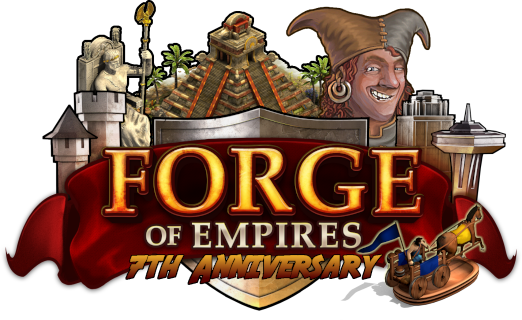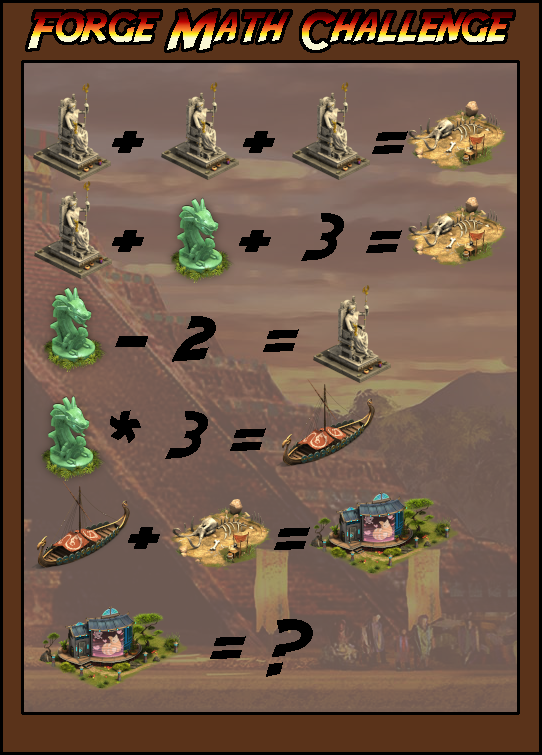# Contest day 6

Status
Not open for further replies.

36

#### os0871

##### Private
The answer is 36.36

#### mandyxxrossi

##### Private
Zeus = 5
Relic = 7
Bones = 15
Boat = 21
VR Dwelling = 36

5 x 3 = 15
5 + 7 + 3 = 15
7 - 2 = 5
7 * 3 = 21
21 + 15 = 36#### Xxsupr

##### Private
First one: 5 + 5 + 5 = 15 (Bones = 15)
Second one: 5 + 7 + 3 = 15 ( Bones = 15 )
Third one: Diamond Relic - 2 = 5 ( Zeus = 5 )
Forth one: Diamond Relic ( 7 ) * 3 = 21 ( Boat = 21 )
Fifth one: Boat ( 21 ) + Bones ( 15 ) = 36 ( Modular VR Dwelling )

So the items are the following numbers:
Zeus = 5
Diamond Relic = 7
Bones = 15

And the answer to the question is:
Boat (21) + Bones(15) = 36 (Modular VR Dwelling)

36

#### Porfuera

##### Private
Hello,

The value of the unknown building/decoration with the cat(?) is: 36

Cheers,

Porfuera.

#### muimui4498

##### PrivateGreetings, Queens and Kings!

2018 brought the Reconstruction Mode to the game, which was one of the most requested features of the game. The research "Mathematics" was needed to unlock it, but now it is on you to use some mathematics to solve the following puzzle!For this contest, 7 players can win 250 goods of your choice! Have fun calculating!

Please submit the correct answer until April 28, 2019. We will pick the 7 lucky winners from all correct answers!Sincerely,
36

Happy Birthday!

Best regards,

36

36

36

#### Attachments

• 467.4 KB Views: 18

#### Timinator2

##### Private

I assigned variables to each object as follows. Zeus = x, skeleton = y, relic = z, ship = a, stage = b. Therefore we end up with the following equations

(1) 3x = y
(2) x + z + 3 = y
(3) z - 2 = x
(4) 3z = a
(5) a + y = b

We can substitute (1) into (2) to get

(6) z + 3 = 2x

We can subtract (3) from (6)

z + 3 = 2x
z - 2 = x
-_________
5 = x

We can plug the value of x back into (1) to find y = 15. Plugging both these values into 3, we find z = 7. Plugging the value of z into (4), we find that a = 21. plugging these values into (5), we find the value of b to be 36.

Therefore, the value of the stage item in the above puzzle is equal to 36.

36

36

36

#### zedeyejoe

##### Private
= 36

Status
Not open for further replies.